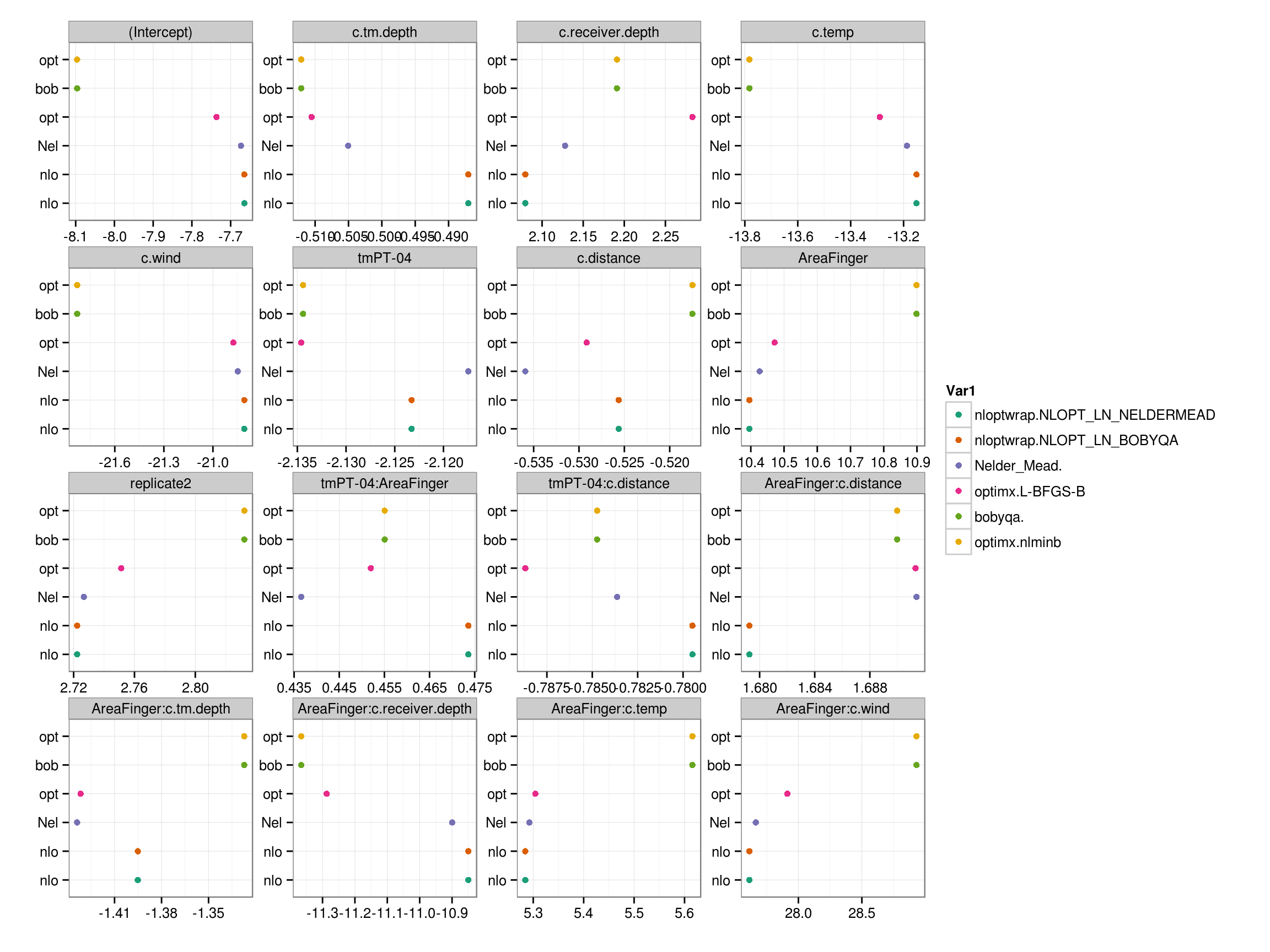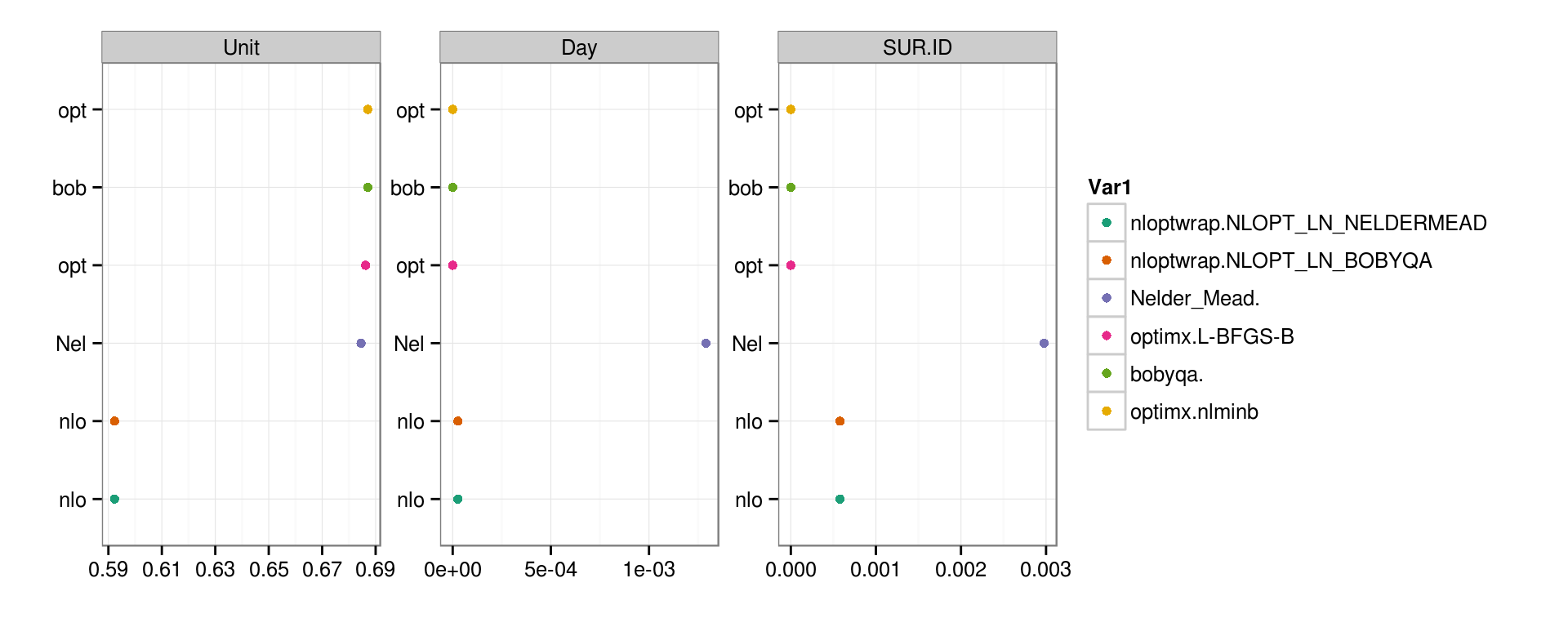# lme4 convergence warnings: troubleshooting

For large data sets and large, complex models (lots of random-effects parameters, or for GLMMs also lots of fixed-effect parameters), it is fairly common to get convergence warnings. (A lot of these were removed in version 1.1-7, but they still happen.) Here I’m going to brain-dump/show a variety of examples of tests that rule out various hypotheses about what’s causing the problem.

• check for singularity
• lower convergence tolerances/run longer
• restart from the ending parameters
• try other optimizers
• consider absolute vs. scaled gradient
library("lme4")
packageVersion("lme4")
##  '1.1.8'
## but should work equivalently with 1.1-7 (latest CRAN version)
library("numDeriv")
library("RCurl") ## to source() from Github
library("ggplot2"); theme_set(theme_bw())
library("reshape2")
library("plyr")
library("RColorBrewer")

## Example

Example from Stack Overflow:

source("SO_23478792_dat.R")
df$SUR.ID <- factor(df$SUR.ID)
df$replicate <- factor(df$replicate)
Rdet <- cbind(df$ValidDetections,df$FalseDetections)
Unit <- factor(1:length(df$ValidDetections)) m1 <- glmer(Rdet ~ tm:Area + tm:c.distance + c.distance:Area + c.tm.depth:Area + c.receiver.depth:Area + c.temp:Area + c.wind:Area + c.tm.depth + c.receiver.depth + c.temp +c.wind + tm + c.distance + Area + replicate + (1|SUR.ID) + (1|Day) + (1|Unit) , data = df, family = binomial(link=logit)) ## Warning in checkConv(attr(opt, "derivs"), opt$par, ctrl =
## control$checkConv, : Model failed to converge with max|grad| = 1.28888 ## (tol = 0.001, component 19) Gets rid of scaling warnings, but we still have a large relative gradient. ## Check singularity If the fit is singular or near-singular, there might be a higher chance of a false positive (we’re not necessarily screening out gradient and Hessian checking on singular directions properly); a higher chance that the model has actually misconverged (because the optimization problem is difficult on the boundary); and a reasonable argument that the random effects model should be simplified. The definition of singularity is that some of the constrained parameters of the random effects theta parameters are on the boundary (equal to zero, or very very close to zero, say $$<10^{-6}$$): tt <- getME(m1_sc,"theta") ll <- getME(m1_sc,"lower") min(tt[ll==0]) ##  0.007956743 Not a problem in this case. ## Double-checking gradient calculations Compare absolute and scaled gradient; compare gradient and Hessian with numDeriv equivalents. We compute the gradient and Hessian in a quick-but-less-accurate way; we can use a more precise (but slower) algorithm implemented by the numDeriv package (Richardson extrapolation). Extract pre-computed information: derivs1 <- m1_sc@optinfo$derivs
max(abs(sc_grad1))
##  1.288884

One general problem is that large scaled gradients are often associated with small absolute gradients: we might decide that we’re more interested in testing the (parallel) minimum of these two quantities:

## control$checkConv, : Model failed to converge with max|grad| = 1.11384 ## (tol = 0.001, component 19) Still have a problem. ## Try a different optimizer Try bobyqa for both phases – current GLMM default is bobyqa for first phase, Nelder-Mead for second phase. We are thinking about changing the default to nloptwrap, which is generally much faster. As we’ll see below, nloptwrap would not help with the convergence warning in this case … m3 <- update(m1_sc,start=ss,control=glmerControl(optimizer="bobyqa", optCtrl=list(maxfun=2e5))) If we set the number of evaluations large enough so the optimization actually finishes, we do avoid the warning. ## Try lots of different optimizers Use this recipe to source the allFit script from Github (it’s also been incorporated into the mixed package): afurl <- "https://raw.githubusercontent.com/lme4/lme4/master/misc/issues/allFit.R" eval(parse(text=getURL(afurl))) ## Loading required package: optimx ## Loading required package: nloptr ## Loading required package: dfoptim aa <- allFit(m2) is.OK <- sapply(aa,is,"merMod") ## nlopt NELDERMEAD failed, others succeeded aa.OK <- aa[is.OK] lapply(aa.OK,function(x) x@optinfo$conv$lme4$messages)
## $bobyqa. ## NULL ## ##$Nelder_Mead.
##  "Model failed to converge with max|grad| = 1.24692 (tol = 0.001, component 19)"
##
## $optimx.nlminb ## NULL ## ##$optimx.L-BFGS-B
##  "Model failed to converge with max|grad| = 1.01503 (tol = 0.001, component 19)"
##
## $nloptwrap.NLOPT_LN_NELDERMEAD ##  "Model failed to converge with max|grad| = 1.27814 (tol = 0.001, component 19)" ## ##$nloptwrap.NLOPT_LN_BOBYQA
##  "Model failed to converge with max|grad| = 1.27814 (tol = 0.001, component 19)"

All but nlminb and built-in bobyqa give convergence warnings.

## So what?

In this case, we can overcome the failure to converge by using a different optimizer. But how bad was the convergence failure, really, in this case? Should we have worried at all?

Log-likelihoods are all approximately the same: the two optimizers that finish withoug convergence warnings have log-likelihoods about 0.07 below the worst fits …

(lliks <- sort(sapply(aa.OK,logLik)))
## nloptwrap.NLOPT_LN_NELDERMEAD     nloptwrap.NLOPT_LN_BOBYQA
##                     -107.1693                     -107.1693
##                     -107.1161                     -107.1130
##                       bobyqa.                 optimx.nlminb
##                     -107.1098                     -107.1098
aa.fixef <- t(sapply(aa.OK,fixef))
aa.fixef.m <- melt(aa.fixef)
models <- levels(aa.fixef.m\$Var1)
ylabs <- substr(models,1,3)
aa.fixef.m <- transform(aa.fixef.m,Var1=factor(Var1,levels=names(lliks)))
(gplot1 <- ggplot(aa.fixef.m,aes(x=value,y=Var1,colour=Var1))+geom_point()+
facet_wrap(~Var2,scale="free")+
scale_colour_brewer(palette="Dark2")+
scale_y_discrete(breaks=models,
labels=ylabs)+
labs(x="",y=""))Coefficients of variation of fixed-effect parameter estimates:

summary(unlist(daply(aa.fixef.m,"Var2",summarise,sd(value)/abs(mean(value)))))
##     Min.  1st Qu.   Median     Mean  3rd Qu.     Max.
## 0.003513 0.017890 0.023470 0.021620 0.028250 0.036460

Not as interesting: all optimizers except N-M give very close to zero std.dev. for Day and SUR.ID

aa.stddev <- t(sapply(aa.OK,function(x) sqrt(unlist(VarCorr(x)))))
print(aa.stddev,digits=3)
##                                Unit      Day   SUR.ID
## bobyqa.                       0.687 1.38e-08 3.73e-08
## optimx.nlminb                 0.687 0.00e+00 0.00e+00
## optimx.L-BFGS-B               0.686 0.00e+00 0.00e+00
## nloptwrap.NLOPT_LN_BOBYQA     0.592 2.66e-05 5.78e-04
aa.stddev.m <- melt(aa.stddev)
aa.stddev.m <- transform(aa.stddev.m,Var1=factor(Var1,levels=names(lliks)))
gplot1 %+% aa.stddev.mI previously tried the lme4.0 package for comparison, but I can’t quite get it to work for various reasons (apparent knitr/caching problems and/or downdated X'X is not positive definite errors): presented here without evaluation.

library("lme4.0")
m5 <- glmer(Rdet ~ tm:Area + tm:c.distance +
c.distance:Area + c.tm.depth:Area +
detach("package:lme4.0")